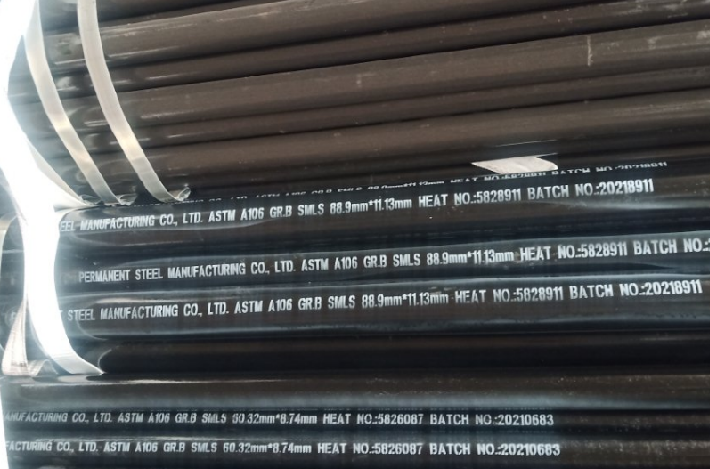Home  >  Hot News  >  Current News

# Seamless Pipe Weight Calculation Formula

Date:2021-11-08    keywords:seamless steel pipe weight calculation formula
Seamless steel pipe weight calculation formula:
1. How to calculate the weight of seamless steel pipe per meter?

Weight per meter of seamless steel pipe: W=0.02466*S(D-S)

Symbol meaning: D: outer diameter S: wall thickness

Example: For a seamless steel pipe with an outer diameter of 60mm and a wall thickness of 4mm, find the weight per meter.
Weight per meter: W=0.02466*4*(60-4)=5.52Kg2. Theoretical weight calculation formula of seamless steel pipe

Seamless steel pipe:  W=0.0246615(D-S)*S
W: Theoretical weight of steel pipe kg/m   D: Nominal outer diameter of steel pipe mm  S: Nominal wall thickness of steel pipe mm

Galvanized steel pipe: W=C[0.02466(D-S)*S]
W: Theoretical weight of the steel pipe kg/m   C: The weight coefficient of the galvanized steel pipe increased compared to the black pipe
D: Black tube outer diameter mm                   S: Black tube wall thickness mm

Example: DN219 seamless steel pipe, wall thickness=6mm

W=(219-6)*6*0.02466=31.51548Kg/M

3. Theoretical weight calculation formula of square tube

kg／m W=[2 (a+b)-4×s]×s×0.00785
In the formula, a and b are the side length of the rectangular tube (mm), and s is the wall thickness (mm)

Example: 30mm×20mm rectangular tube, wall thickness 1mm, find the weight per meter
Weight per meter = [2×(30+20)-4]×1×0.00785 kg =0.7536kg

Galvanized square tube:

kg／m W=[2 (a+b)-4×s]×s×0.00785x1.06